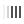# Calculator conversion of kilowatts to horsepower (kW to hp)

The current calculator, performing the conversion by the engine power multiplying, expressed in kW, by a multiplier of 1.3596 (i.e., while using a conversion coefficient of 1 hp. = 1.35962 kW), converts the car horsepower to the power expressed in kW, while using common coefficient.

## How many kilowatts are contained in one horsepower and vice versa

• 1 kW = 1.3598 hp (for metric calculation);
• 1 kW = 1.3783 hp (English standard);
• 1 kW = 1.34048 hp (electric «horse»).

So, there are several measurement units called «horsepower», but, we mean “metric horsepower”, equal to ≈0.7354 kW, as a rule. But both United States and Great Britain equate automobile-related horsepower to 0.7456 kW, i.e., like 75 kgf • m/s, which is approximately 1.0138 metric. If converting the power of 1 horsepower per kilowatt in industry or energy, it will be equal to ≈0.746. Therefore, decide what kind of reference horse you need to choose before using our power converter kW to hp in order to get the accurate result.

## The way to use the power converter from kW into hp

1. It’s required to choose one of three standards, first of all, in order to convert «horsepower to kilowatts» or vice versa.
2. Then select the unit to convert the kW/W or hp into.
3. Enter the value in the field you are going to convert.

## FAQ

• ### How many kilowatts are in one horsepower?

One metric horsepower equals 0.735 kilowatts (745.7 watts) when calculating engine power in kilowatts. How many kilowatts are in 1 hp is calculated using the formula: power in kilowatts divided by a factor of 1.36.

• ### How many hp in kW?

To convert horsepower to the unit of kilowatts, you need to multiply its value by 0.735.

• ### How much is 5 hp in kW?

According to the metric calculation, where the ratio of 1kW equals 1.359 hp. — 5 horsepower is approximately equal to 3.68 kilowatts of power. If the calculation is done with an electric motor, then 5 hp would be 3.73 kW, since the ratio where 1kW = 1.34048 hp should be used when running an electric motor.

• ### 7 kW is how much in hp?

7 kW would be equal to 9.5 hp. The ratio 1 kW = 1.359 hp is used to get this conversion, so the power in kilowatts is multiplied by a factor of 1.36.

Loading comments